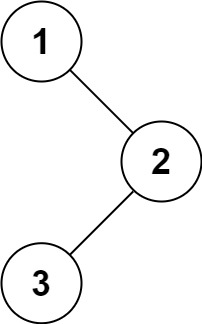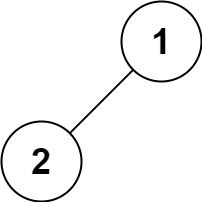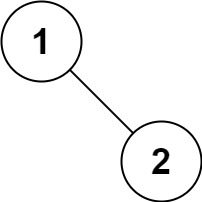# LeetCode | 144. Binary Tree Preorder Traversal

Given the root of a binary tree, return the preorder traversal of its nodes' values.

Example 1:Input: root = [1,null,2,3]
Output: [1,2,3]


Example 2:

Input: root = []
Output: []


Example 3:

Input: root = 
Output: 


Example 4:Input: root = [1,2]
Output: [1,2]


Example 5:Input: root = [1,null,2]
Output: [1,2]


Constraints:

• The number of nodes in the tree is in the range [0, 100].
• -100 <= Node.val <= 100

Recursive solution is trivial, could you do it iteratively?

/**
* Definition for a binary tree node.
* struct TreeNode {
*     int val;
*     TreeNode *left;
*     TreeNode *right;
*     TreeNode(int x) : val(x), left(NULL), right(NULL) {}
* };
*/
class Solution {
public:
void backPreorder(TreeNode* root, vector<int>& res) {
if(root == NULL)
return;
res.push_back(root->val);
backPreorder(root->left, res);
backPreorder(root->right, res);
return;
}
vector<int> preorderTraversal(TreeNode* root) {
vector<int> res;
backPreorder(root, res);
return res;
}
};

03-023260
09-282801-253万+
04-232162
03-194027
05-256613
11-121968
05-23131
03-2310万+
01-0415万+